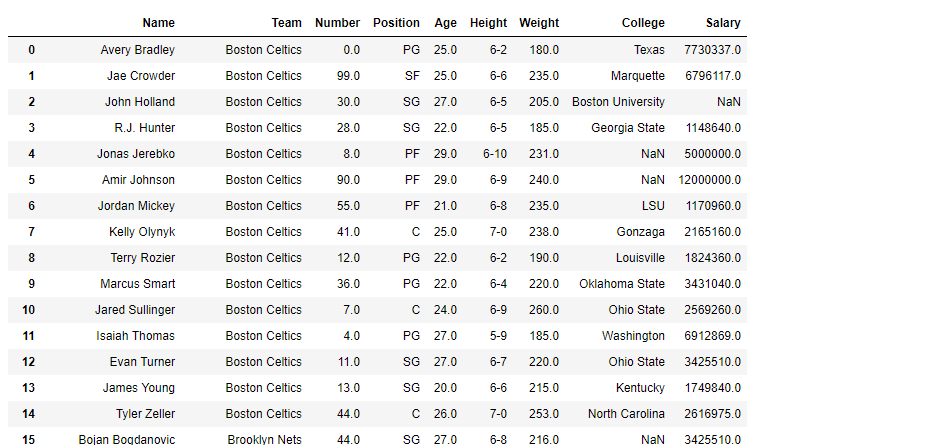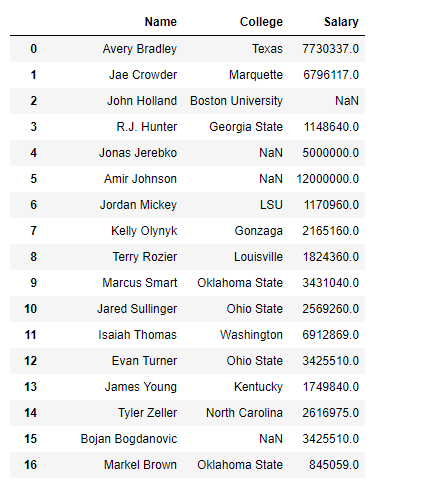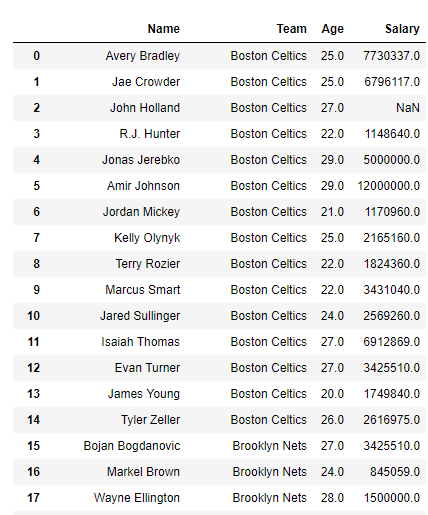Open in App
Not now

# Python | Pandas dataframe.filter()

• Difficulty Level : Expert
• Last Updated : 19 Nov, 2018

Python is a great language for doing data analysis, primarily because of the fantastic ecosystem of data-centric python packages. Pandas is one of those packages and makes importing and analyzing data much easier.

Pandas` dataframe.filter()` function is used to Subset rows or columns of dataframe according to labels in the specified index. Note that this routine does not filter a dataframe on its contents. The filter is applied to the labels of the index.

Syntax: DataFrame.filter(items=None, like=None, regex=None, axis=None)

Parameters:
items : List of info axis to restrict to (must not all be present)
like : Keep info axis where “arg in col == True”
regex : Keep info axis with re.search(regex, col) == True
axis : The axis to filter on. By default this is the info axis, ‘index’ for Series, ‘columns’ for DataFrame

Returns : same type as input object

The items, like, and regex parameters are enforced to be mutually exclusive. axis defaults to the info axis that is used when indexing with [].

Example #1: Use `filter()` function to filter out any three columns of the dataframe.

 `# importing pandas as pd``import` `pandas as pd`` ` `# Creating the dataframe ``df ``=` `pd.read_csv(``"nba.csv"``)`` ` `# Print the dataframe``df`Now filter the “Name”, “College” and “Salary” columns.

 `# applying filter function ``df.``filter``([``"Name"``, ``"College"``, ``"Salary"``])`

Output :Example #2: Use `filter()` function to subset all columns in a dataframe which has the letter ‘a’ or ‘A’ in its name.

Note : `filter()` function also takes a regular expression as one of its parameter.

 `# importing pandas as pd``import` `pandas as pd`` ` `# Creating the dataframe ``df ``=` `pd.read_csv(``"nba.csv"``)`` ` `# Using regular expression to extract all``# columns which has letter 'a' or 'A' in its name.``df.``filter``(regex ``=``'[aA]'``)`

Output :The regular expression ‘[aA]’ looks for all column names which has an ‘a’ or an ‘A’ in its name.

My Personal Notes arrow_drop_up